12
1Department of Radiation Physics, The University of Texas MD Anderson Cancer Center, Houston, Texas, USA.
2Department of Medical Physics, Mary Bird Perkins Cancer Center, Baton Rouge, Louisiana, USA.

Cite this article as: Wang H, Vassiliev ON. Microdosimetric characterisation of radiation fields for modelling tissue response in radiotherapy. Int J Cancer Ther Oncol 2014; 2(1):020116.
DOI:
http://dx.doi.org/10.14319/ijcto.0201.16

Original Article

Abstract

Purpose: Our overall goal is the development of an approach to model tissue response to radiotherapy in which a tissue is viewed as a statistical ensemble of interacting cells. This involves characterisation of radiation fields on the spatial scale of subcellular structures. On this scale, the spatial distribution of radiation energy imparted to tissue is highly non-uniform and should be characterised in statistical terms. Microdosimetry provides a formalism developed for that purpose. This study addresses limitations of the standard microdosimetric approach to modelling tissue response by introducing two new characteristics that include additional information in a form convenient for this application.

Methods: The standard microdosimetric approach is based on the concept of a sensitive volume (SV) representing a target volume in the cell. It is considered in isolation from other SVs, implying that energy depositions in different SVs are statistically independent and that individual cells respond to radiation independent of each other. In this study, we examined the latter approximation through analysis of correlation functions. All calculations were performed with Geant4-DNA Monte Carlo code.

Results: We found that for some realistic scenarios, spatial correlations of deposited energy can be significant. Two new characteristics of radiation fields are proposed. The first is the specific energy-volume histogram (zVH), which is a microscopic analogue of the dose-volume histogram. The second describes the probability distribution of deposited energies in two SVs without assuming statistical independence between the SVs. Numerical examples for protons and carbon ions of therapeutic energies are presented and discussed.

Conclusion: We extended the microdosimetric approach to modelling tissue response by including additional important characteristics and presented them in a more conventional radiotherapy format.

Keywords: Tissue response; Microdosimetry; Hadron therapy

Introduction

Microdosimetry plays an important role in radiation therapy research. The microdosimetric approach to modelling the response of biological systems to radiation has been particularly useful in hadron therapy. Several studies have applied it to calculating the relative biological effectiveness (RBE) of therapeutic proton and carbon beams.1-8 Furthermore, microdosimetric techniques are indispensable for evaluating dose equivalent outside the treatment field, including neutrons.9-11 The microdosimetric approach is also applicable to modelling the biological effects of sparsely ionizing radiations. It has been applied to RBE analysis of radiation sources used in brachytherapy 12-14 as well as external beam therapy with photon and electron beams.15, 16 Microdosimetric concepts are also a foundation of several models of cell survival, for example.17-22

Nevertheless, microdosimetry has had a rather limited impact on clinical practice. It is not mentioned in a recent task group report from the American Association of Physicists in Medicine on biologically related models for treatment planning.23 Part of the reason for this omission is the lack of a fully established methodology for applying microdosimetry to radiotherapy. For example, the question of whether single-event microdosimetric spectra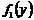(where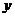is the lineal energy) or multiple-event spectra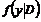should be used at the relatively high dose levels typical for radiotherapy has been subject to debate.24, 25 Another unsettled matter is the size of the sensitive volume (SV). Usually a size on the order of 1 mm is used, but this number has been constantly challenged. A recent study 22 strongly suggested that for modelling cell survival, the SV size should be much smaller, about 10 nm.

There is another problem that, to the best of our knowledge, has not yet been addressed. The standard assumption in microdosimetry is that the response of a biological system, such as the number of surviving cells, can be deduced from a microdosimetric spectrum for a single SV. This implies that energy deposition in a given SV is statistically independent from energy depositions in other SVs, either within the same cell or in neighbouring cells, and that individual cells respond to radiation independent of one another. In the present study, we report data showing strong correlations between energy depositions in two SVs that do not attenuate with increasing distance between the SVs, up to 10 mm (the maximum distance that we tested).

As for the cellular response, it is well known that cells in tissues are not independent. In fact, radiation-induced bystander effects have received much attention in recent years.26 The bystander effect, however, is outside the scope of the present study, which is limited to the physics only. Furthermore, although the definition of a microdosimetric spectrum includes the probability of no energy deposition in an SV 27, this probability is rarely reported or considered in radiobiological models. In hadron therapy, especially for carbon beams, it is significant even for the relatively large (on the cellular scale) volumes on the order of 1 mm3. This probability characterises the spatial heterogeneity of energy deposition on the microscopic scale and, therefore, is an important parameter that should be considered, especially when modelling the tumour control probability (TCP). To address these problems, we introduce in this study new parameters for characterising statistical properties of microscopic patterns of energy deposition. These parameters are similar to the standard microdosimetric quantities 27 but include additional information in a form convenient for modelling tissue response in radiotherapy.

Methods and Materials

This study is based on Monte Carlo simulations performed with Geant4-DNA, release 9.6 (May 17, 2013). The physics implemented in the code is well described elsewhere.28 The physics models needed for the present study are presently available only for one material, liquid water. This is one of the standard materials that is considered tissue equivalent for the purposes of radiobiological modelling. For modelling direct interactions of radiation with cellular targets, it would be preferable to use physics data, such as interaction cross sections, that are specific for each target type (e.g., a DNA molecule). Such data presently is not available owing largely to the complexity of the physics of such interactions, especially at the very low energies that are important for this study. The interaction processes included in the model, for all particles that we simulated, and the respective energy ranges in which the process is active, are as follows:

• Electrons: elastic scattering (7.4 eV-1 MeV), electronic excitation (9 eV-1 MeV), ionization (11 eV-1 MeV), vibrational excitation (2 eV-100 eV), attachment (4 eV -13 eV)
• Protons: electronic excitation (10 eV-100 MeV), ionization (100 eV-100 MeV), electron capture (100 eV-100 MeV)
• Hydrogen atoms: electronic excitation (10 eV-500 keV), ionization (100 eV-100 MeV), charge increase (100 eV-100 MeV)
• Carbon ions: ionization (0.5 MeV/u-106 MeV/u); the model does not include electronic excitations or charge exchange processes (electron capture or loss), and therefore the results we present for carbon beams should be viewed qualitatively only.

Charge transfer processes, electron capture for protons, and charge increase for hydrogen atoms become important at very low energies, near the track end. They become the dominant inelastic processes at particle energies lower than approximately 100 keV.29 In electron capture processes, an electron from a water molecule is transferred to the incident proton to form a neutral hydrogen atom. Conversely, in the charge increase processes, the electron is stripped from the neutral hydrogen atom and, normally, is ejected in a forward direction with approximately the same velocity as the incident hydrogen atom.29 Charge transfer processes are no less important for low-energy carbon ions. In this case, however, the physics is much more complex, in part because a carbon ion can be in any of several charge states, from a fully stripped ion (charge +6) to a neutral atom. These processes are not included in the current version of Geant4-DNA. We will add that the number of proton therapy centers significantly exceeds the number of active carbon therapy facilities. Therefore, results for proton beams are more important at the present time.

Electron histories were terminated when their kinetic energy fell below 11 eV (the ionization threshold in the model). The residual range of an 11 eV electron is on the order of 10 nm according to Francis et al. 30 However, this number is associated with high uncertainty. Nikjoo and Lindborg 31, for example, reported that the range of a 100 eV electron is only 4.9 nm. Terminating electron history at 11 eV was unlikely to introduce significant errors in our study because the spatial resolution of the results we reported was 10 nm. Histories of protons and carbon ions were terminated after they travelled a distance of about 10 mm. Therefore, no energy cutoffs were applied to these particles.

Energy depositions were tallied in a box sized 1 mm x 1 mm in the X and Y directions and 10 mm in the Z direction. The initial direction of particle travel was parallel to the Z axis. The size of the simulation volume exceeded that of the tally box by 20 nm in all directions. The 20 nm of padding was needed to achieve equilibrium of secondary electrons produced by protons and carbon ions throughout the tally box, which was estimated using Monte Carlo simulations. The material of the simulation volume was liquid water. The tally box was divided into 10 nm cubic voxels. Energy depositions were tallied separately for individual voxels.

A parallel beam of particles travelling in the positive Z direction was incident on one side of the simulation volume. The beam size was 1.04 mm x 1.04 mm, which coincides with the size of the simulation volume in the XY direction. The number of incident particles in one history was randomly sampled from a Poisson distribution. The average number of incident particles per history (parameter of the Poisson distribution) was chosen so that the average dose in the tally box was 2 Gy per history. This value (2 Gy) is representative of a typical dose delivered to the tumor volume in one treatment fraction. In hadron therapy, the dose is usually prescribed in Gy (RBE). In this study, however, we specified the absorbed dose in Gy only. Using Gy (RBE) would require specifying an RBE value, which, conceptually, would contradict our overall purpose of improving tissue response models.

The following quantities were calculated:

• Probability distributionof deposited energy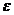in individual 10 nm voxels. This included the probability of no energy deposition,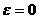. The energy resolution was 1 eV. Generally,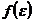depends on the dose and should be written as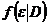. However, all results reported in this study are at the same dose of 2 Gy, and therefore,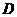is omitted throughout the text.
• The correlation function in the Z direction,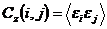, whereand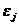are energies deposited in voxels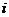and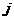, both located on a line parallel to the Z axis. Owing to the symmetry in the XY plane within the tally box (parallel beam), the functiondoes not depend on X or Y.
• The correlation function in the lateral direction,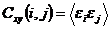, where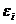andare energies deposited in voxelsand, both of which are located in a plane orthogonal to the Z axis. Calculations were performed separately for several depths, from 0 to 10 mm.
• The probability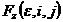of energy deposited in each of the two voxelsandexceeding, with both voxels located on a line parallel to the Z axis. Like the correlation functions, this quantity characterises spatial correlations of energy depositions.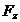, however, is more convenient for radiobiological modelling. We did not calculate this function for the lateral direction because our data showed that correlations in that direction were very short-range and therefore negligible. In that case, in the lateral direction, this probability can be found from distributionsfor individual voxels as a product of probabilities.

The version of Geant4-DNA that is available for download does not include code that calculates the above quantities. For the purposes of this study, we wrote a new user code, the main parts of which were a new source subroutine and a subroutine for tallying the quantities of interest. In Geant4 terminology, these are referred to as the "primary generation action" and "event action", respectively. The physics models that we included were consistent with the "microdosimetry" example included in the standard installation of Geant4. Energy deposition correlation functions were introduced based on the previous work of one of the authors on the application of Monte Carlo techniques to problems of statistical mechanics.32,33

Results and Discussion

Energy deposition patterns at 2 Gy

Figures 1 and 2 show typical energy deposition patterns at 2 Gy in a 1 mm cube for 10 MeV and 100 MeV protons. No neutral hydrogen atoms were produced in these examples. As shown in the figures, energy deposition events were mostly localized very close to proton tracks. Only in relatively rare events were delta electrons produced with energies high enough to travel a distance of more than a few nanometers away from a proton track. The total number of energy deposition events is approximately the same in the two figures. At 10 MeV, as expected, the number of energy deposition events per 1 mm track segment was much higher than that at 100 MeV. At 10 MeV, a notably large fraction of the volume was not affected directly by protons or delta electrons.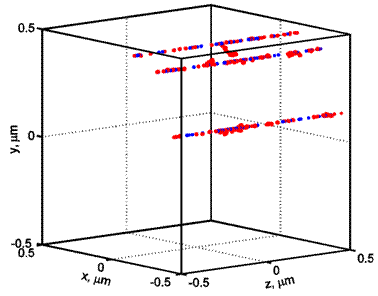FIG.1: An energy deposition pattern for 10 MeV protons. The number of tracks shown is typical for a dose of 2 Gy. Each dot represents an energy deposition event (e.g., ionization or excitation). Proton tracks are shown in blue, and delta electron tracks are shown in red.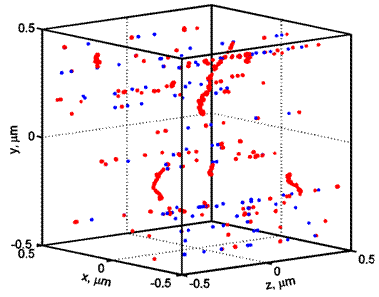FIG. 2: An energy deposition pattern for 100 MeV protons. The number of tracks shown is typical for a dose of 2 Gy. Each dot represents an energy deposition event (e.g., ionization or excitation). Proton tracks are shown in blue, and delta electron tracks are shown in red.

Carbon tracks of therapeutic energies (not shown) are characterised by an even higher number of energy deposition events per micrometer than 10 MeV protons. Accordingly, the average number of carbon ions needed to deliver a 2 Gy dose is lower. For example, for a carbon beam with an energy of 100 MeV/u, at 2 Gy, the average number of carbon ions entering the tally volume (1 mm x 1 mm x 10 mm) is 0.48. This estimate was derived using the total stopping power calculated using SRIM software.34 Physical dose per fraction in carbon therapy is usually lower than 2 Gy by a factor of approximately 2-3 because of the high RBE. The average number of tracks in a volume of this size is thus lower by the same factor.

Probability distribution of energy deposited in 10 nm voxels

All voxels in the tally volume had approximately the same probability distribution of deposited energybecause the incident beam was parallel and its properties did not change significantly as it propagated the 10 mm distance. Figure 3 shows a histogram estimate offor 10 MeV and 100 MeV protons, as well as 100 MeV/u carbon ions. The width of each bar in the figure is 1 eV. In this figure, the distribution is normalized as follows: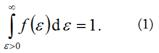This means thatis a conditional distribution given the deposited energy is greater than zero.

The first bar represents energy depositions of less than 1 eV, excluding zero. These result from excitations of vibrational degrees of freedom of a water molecule by delta electrons. The distinct peaks seen in the figure correspond to discrete levels of electronic excitations. As is evident in the figure, 10 MeV protons had a higher probability of depositing energy greater than 34 eV than did 100 MeV protons, and carbon ions were more likely to deposit energy greater than 56 eV than were protons. It should be mentioned that this is a conditional probability given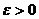. The mean deposited energies were 47.6 eV, 38.9 eV, and 37.2 eV for 10 MeV, 50 MeV, and 100 MeV protons, respectively. The corresponding standard deviations were 62.1 eV, 56.0 eV, and 54.9 eV. These results are usually expressed in terms of the frequency mean lineal energy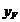, and dose mean lineal energy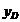. It should be noted, however, thatandare normally used to characterise a single event distribution, when a track of only one particle interacts with the SV. Our distributions are multiple-event, that is, the number of particles interacting with a voxel is random and can be greater than 1. Fortunately, for the 10 nm voxels, at 2 Gy, the most likely number of particles is 0 or 1. More specifically, the probability of an individual 10 nm voxel receiving a nonzero energy was 2.57 x 10-4,  3.10 x 10-4, and 3.27 x 10-4 for protons of energies 10 MeV, 50 MeV, and 100 MeV, respectively. This leaves a large number of voxels not affected directly by radiation, and, on the other hand, very few voxels will be hit more than once. The calculatedwere 7.15 eV/nm, 5.83 eV/nm, 5.58 eV/nm, andwere 19.3 eV/nm, 17.9 eV/nm, and 17.7 eV/nm for 10 MeV, 50 MeV, and 100 MeV protons, respectively. Chmelevsky and Kellerer 35 reported similar results and also used Monte Carlo simulations. For 5 MeV and 20 MeV protons, they reportedof approximately 10.8 eV/nm and 8.62 eV/nm (read from a graph), andof 20.3 eV/nm and 17.4 eV/nm. The SV was a sphere of 10 nm diameter. For the same SV and 20 MeV protons, a figure in the ICRU Report 36 27 showsof approximately 17.8 eV/nm. Ouragree with the previous studies, whereas ouris somewhat lower. The latter is known to be more sensitive to the radiation transport model. In Chmelevsky and Kellerer 35 only ionizations were accounted for. This resulted in higher energy per event because ionizations are associated with higher energy deposits than excitations, either electronic or vibrational.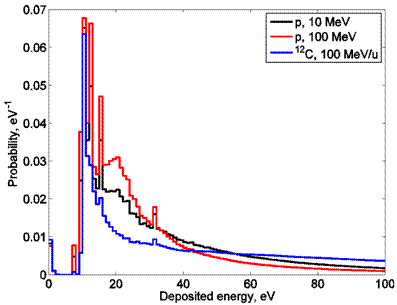FIG. 3: Probability distribution of energy deposited in a 10 nm voxel at 2 Gy for 10 MeV and 100 MeV protons, and for 100 MeV/u carbon ions.

Specific energy-volume histogram (zVH)

In radiotherapy, the dose-volume histogram (DVH) is routinely used to describe 3D dose distributions. Here we applied the same idea to microscopic distributions of radiation energy imparted to tissues. We introduce the specific energy-volume histogram, zVH. The specific energyis defined as the quotient of energydeposited in an SV by the mass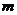of tissue in it. 27 Specific energy has the same units as the absorbed dose (Gy). We define zVH as the average total volume V occupied by voxels receiving a specific energy greater than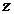. The zVH is a function ofand depends on the dose and voxel size.

The latter means that introducing the zVH does not completely solve the problem of choosing an appropriate SV size. However, the zVH somewhat mitigates this problem because the voxel has a different meaning than the SV. The voxel defines the resolution of spatial discretization. Furthermore, 10 nm is likely an optimal size for a voxel. Choosing a larger size would result in a lower spatial resolution and loss of information about interactions on a nanoscale. Voxels smaller than 10 nm would certainly provide a more detailed description of the physical stage of radiation effects on tissues. However, the physical stage is followed by the chemical stage, which is associated with delocalization of deposited energy through the diffusion of water radiolysis products that travel distances exceeding a few nanometers.36 Considering the computational cost and large uncertainties in the cross-sectional data at low energies near the end of electron track, the benefits of a further reduction of the voxel size are questionable.

Calculated zVH for 10 MeV and 100 MeV protons, and 100 MeV/u carbon beams are shown in Figure 4. Compared with the DVH, the shape of the zVH resembled DVH for organs at risk. However, the scales of both axes differed considerably. Although the dose shown in Figure 4 was only 2 Gy, in terms of the specific energy, all three curves extended beyond 40 kGy. At the same time, the figure shows that only a very small fraction of tissue volume received any energy directly from either proton or carbon beams. Qualitatively, this result is consistent with the energy deposition patterns shown in Figures 1 and 2. Finally, at exactly the same dose for the all three beams, the three zVH curves were distinctly different. Given that the biological effects would also be different, this suggests that models can be developed that correlate properties of the zVH with tissue response. For example, similar to the standard approach used in microdosimetry 24, the biological effect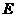at a given dosecan be represented as a linear functional of the zVH: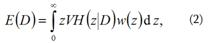where function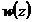describes the response of a biological system and can be determined by fitting Eq. (2) to a set of experimental data for different radiation fields. Alternatively, or in addition to Eq. (2), simple metrics associated with the zVH can be tested for correlation with tissue response. Such metrics based on the DVH are used routinely in radiotherapy for evaluating treatment plans and predicting treatment outcomes. By analogy to the metrics used in radiotherapy, metrics based on the zVH may include, for example, V (20 kGy), that is, the volume receiving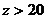kGy. Compared with the dose dependent multiple-event microdosimetric spectrum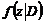, the zVH presents data in a format more familiar to radiotherapy professionals and clearly shows the volume not affected directly by radiation (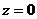). The RBE can be found from Eq. (2) simply as the ratio of doses that produce the same effect, that is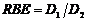, where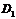and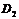satisfy equation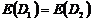.

Correlation functions

Figure 5 shows correlation functions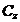for energy deposited in two voxels located on a line parallel to the Z axis. The data shown are for 10 MeV and 100 MeV proton beams, and a 100 MeV/u carbon beam. As illustrated in the figure, all three curves were very flat, which indicates that correlations did not attenuate over distances exceeding 10 mm. Clearly, correlations remain strong at distances on the order of the distance between nuclei of two neighbouring cells, and likely at even larger distances, which results in an increased probability of a proton or especially a carbon ion producing a cluster of damaged cells along its track. This effect may alter how the affected cells respond to radiation and, therefore, should be considered when modelling tissue response. The spatial resolution of the data was 10 nm. Small fluctuations in the data were due to the statistical uncertainties of Monte Carlo simulations. The spike at the graph origin represents self-correlation,, where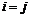. All three self-correlation values shown in Figure 5 are consistent with the standard deviations of deposited energies reported earlier.

In contrast, correlations in the lateral direction attenuate very rapidly. This can be seen in Figure 6, which shows the correlation function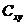. To estimate the correlation radius,,as a function of distance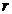between two voxels was approximated through a least squares fit by the exponent. The best-fit values of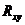for 10 MeV, 50 MeV, and 100 MeV protons were 12.3 nm, 11.4 nm, and 10.8 nm, respectively. For 100 MeV/u carbon ions, theestimate was 12.8 nm. Apparently, the correlation radius tends to increase with increasing LET. The fit was limited to a distance of less than 100 nm. Beyond 100 nm, the correlation function approached its asymptotic value, indicated in the figure by a horizontal dashed line. This value represents a complete attenuation of correlations, that is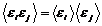. The asymptotic value was the same for all three beams shown because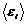is proportional to the dose, which was the same (2 Gy) for all three beams. The asymptotic value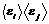for the dose of 2 Gy was calculated completely independently from the Monte Carlo simulations. Finally, the statistical uncertainties were rather large at large distances because of a small probability of having non-zero deposited energies in both voxels.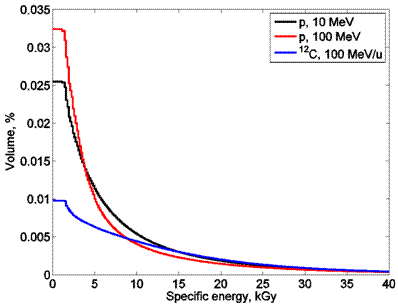FIG. 4: The specific energy-volume histogram, zVH, for 10 MeV and 100 MeV protons and for 100 MeV/u carbon ions.FIG.5: Correlation functions in the direction parallel to particle tracks (Z axis),, for 10 MeV and 100 MeV protons and for 100 MeV/u carbon ions.

Probability distribution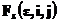Similarly to the correlation function, the probability distribution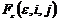characterises correlations of energy deposition events along the direction of a particle track. Given that correlations in the lateral direction are virtually nonexistent, such characterisation is adequate for modelling tissue response. The advantage ofis that it explicitly specifies the probabilities of two voxels receiving a certain amount of energy. This information can be correlated with tissue response through a model (for example, phenomenological) similar to the one given by Eq. (2). Alternatively, the more conventional joint probability distribution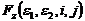could be used for that purpose. However, it is less convenient because it has an extra argument, and we do not anticipate that the extra information this argument provides will profoundly improve the model.

Figure 7 shows the distribution, with solid lines for two voxels separated by a distance of 1 mm, for 10 MeV and 100 MeV proton beams and for a 100 MeV/u carbon ion beam. We found that the distribution did not depend on the distance between two voxels of up to a distance of 10 mm, which was the maximum distance that we tested. This is consistent with the behaviour of the correlation functions discussed earlier. As shown in the figure, the probability of each of the two voxels receiving high energy increased with increasing LET. Furthermore, to demonstrate the profound effect correlations have on this distribution, we also calculated it assuming that the two voxels are completely independent and using the distribution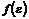for an individual voxel. This result is shown in Figure 7 with dashed lines. These calculated probabilities were orders of magnitude lower. Therefore, to simplify comparisons, we show these probabilities multiplied by a factor of 102, 103, and 104 for 100 MeV protons, 10 MeV protons, and 100 MeV/u carbon ions, respectively. Clearly, assuming statistical independence among voxels may result in significant errors, even when voxels are separated by a large, on the cellular scale, distance of 10 mm.

A comparison of Figure 7 and Figure 4 shows that the order of the three curves near the origin was different. This is because the data shown in Figure 7 were a result of the interplay of two factors. The first factor is the probability of a nonzero energy deposit in the first of the two voxels, the maximum of which occurs for 100 MeV protons, as is shown in Figure 4. The second factor is the conditional probability of a nonzero energy deposit in the second voxel, given that a nonzero energy was deposited in the first voxel. This conditional probability is maximal for carbon beams because they show a stronger correlation (Figure 5).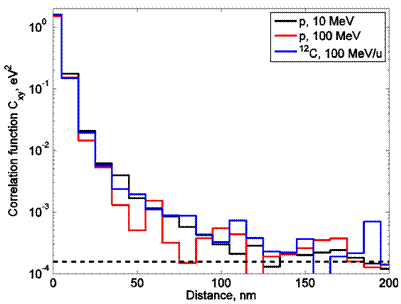FIG. 6: Correlation functions in the lateral direction with respect to particle tracks (XY plane),, for 10 MeV and 100 MeV protons and for 100 MeV/u carbon ions. The horizontal dashed line indicates the asymptotic value, which represents a complete attenuation of correlations at large distances.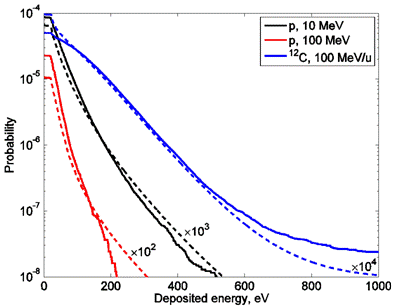FIG. 7: The probabilityof energy deposited in each of the two voxelsandexceeding(solid lines). Both voxels are located on the Z axis and separated by a distance of 1 mm. Data are shown for 10 MeV and 100 MeV protons and 100 MeV/u carbon ions. The dashed lines show the same probability calculated assuming statistical independence. This assumption results in underestimation by orders of magnitude. Thus, to simplify comparison, these results are shown multiplied by a factor of 102, 103, and 104, as indicated in the figure.

Conclusion

On the spatial scale of subcellular structures, radiation fields are rather complex and should be described in statistical terms. Microdosimetry has a formalism developed for that purpose. However, the amount of detail needed to predict tissue response in radiotherapy remains unclear. In this study, we extended the microdosimetric approach somewhat to include characteristics that may be important for that application and to present them in a more conventional radiotherapy format. We have shown that in some realistic scenarios, both the spatial correlations of deposited energy and the volume not directly affected by radiation can be significant. We hypothesize that these variables may be important when modelling normal tissue complications or tumor control. This may be particularly important considering the growing evidence indicating that cells in tissues do not respond to radiation independent of one another. If, for example, spatial correlations in radiation fields indeed result in the formation of clusters of damaged cells, the tissue may respond differently than it would if damaged cells were spread more uniformly throughout the tissue. All examples presented in this paper concern hadron therapy because the effects described herein are more prominent, and the radiobiology of tissue response more directly affects treatment optimization and, for this reason, remains a very active area of research.

Conflict of Interest

The authors declare that they have no conflicts of interest. The authors alone are responsible for the content and writing of the paper.

Received December 31, 2013; Revised February 07, 2014; Accepted February 19, 2014; Published Online February 23, 2014

Corresponding author: : Oleg N Vassiliev, PhD; 4950 Essen Lane, Baton Rouge, LA, 70809, USA. Email: ovassiliev(at)marybird.com